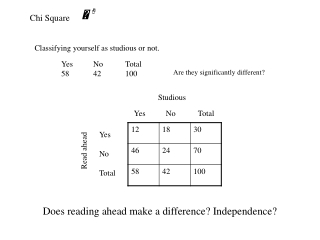DownloadDownload PresentationChi Square

# Chi Square

Télécharger la présentation## Chi Square

- - - - - - - - - - - - - - - - - - - - - - - - - - - E N D - - - - - - - - - - - - - - - - - - - - - - - - - - -
##### Presentation Transcript

1. Chi Square Classifying yourself as studious or not. Yes No Total 58 42 100 Are they significantly different? Studious Yes No Total Yes No Total Read ahead Does reading ahead make a difference? Independence?

2. One variable Choice of PSYA01 Section L01 L02 L03 L30 Total 25 40 15 36 116 Is this more than a chance difference? O = the observed frequency in a category E = the expected frequency in that category We may expect different categories to have the same frequency if chance alone is at work. = .55 + 4.17 + 6.79 + 1.69 = 13.17 Is this significant? Go to the table. df = k - 1

3. Two Variable Are the two variables independent of each other? Contingency Table Career Choice Nat. Sci. Soc. Sci Totals contingency is another word for “possibility” Male Female Totals So this is a “table of possibilities” Marginal Totals The key is determining the expected frequencies of the four observed frequencies (the 4 colored cells).

4. Two Variables – Expected Frequencies Testing the null hypothesis that the variables are independent We know that the probability of the joint occurrence of two independent events is the product of their separate probabilities. e.g., (84/162) X (53/162) = .1696 or 16.96% of the observations are expected in the upper left hand cell. But, N (162) times = 27.48 (expected frequency) Expected Frequencies Now we can use…..

5. Expected Frequencies and Alternative Calculations R = the row total C = the column total = 3.30 + 3.55 + 1.60 + 1.78 = 10.18 Is the probability of this Chi-Square value (or larger) less than .05?

6. Degrees of Freedom for Two Variables df = (R-1)(C-1) R = the number of rows C = the number of columns With our example: df = (2-1)(2-1) = 1 Go to Chi-Square Table and you find that the critical value is 3.84. Our Chi-Squared obtained must be larger than 3.84 for us to reject the null hypothesis. What was the null hypothesis?

7. Phi Coefficient Will establish (at the .05 alpha level) whether two variables are related. A significant Chi-Square means we reject the null hypothesis (which assumes that the two variable are independent. We feel we have evidence That the two variable are related. Gives the numerical value to the relation. The value can range from zero to one. Zero meaning no relation at all (independence) and one indicating a prefect relations. If you know one variable’s value you, you can perfectly predict the value of the other variable.